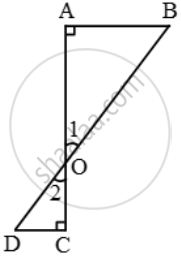# In figure, if ∠A = ∠C, then prove that ∆AOB ~ ∆COD - Mathematics

Sum

In figure, if ∠A = ∠C, then prove that ∆AOB ~ ∆COD#### Solution

In triangles AOB and COD, we have

∠A = ∠C [Given] and, ∠1 = ∠2   [Vertically opposite angles]

Therefore, by AA-criterion of similarity, we have

∆AOB ~ ∆COD

Concept: Similarity
Is there an error in this question or solution?